Home » Handle NULL values in Power Query

# Handle NULL values in Power Query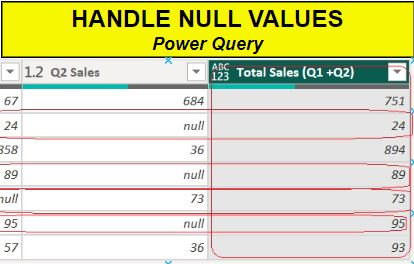This article demonstrate how to handle NULL values in data. Having null values in data may impact calculations and produce wrong result.

As you can see, here we have one table and the column price having few NULL values.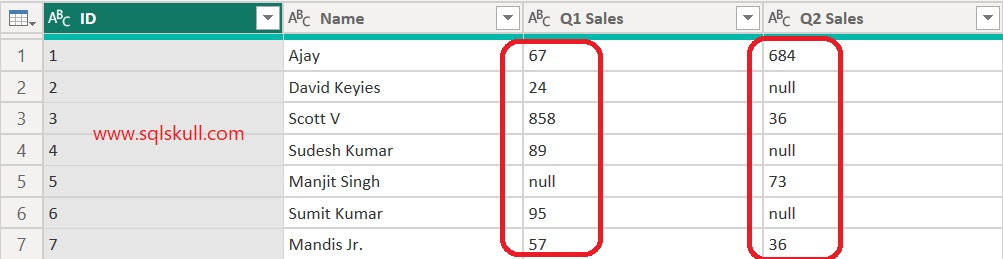Let’s see, how null values may impact the result. Suppose you have to calculate the total sales that is Q1 Sales + Q2 sales in Power Query M code.

For this you simply create a custom column and write below M code.

`Total Sales (Q1+Q2) = [Q1 Sales] + [Q2 Sales]`

This code is perfectly fine and returns the total sales by adding Q1 and Q2 sales.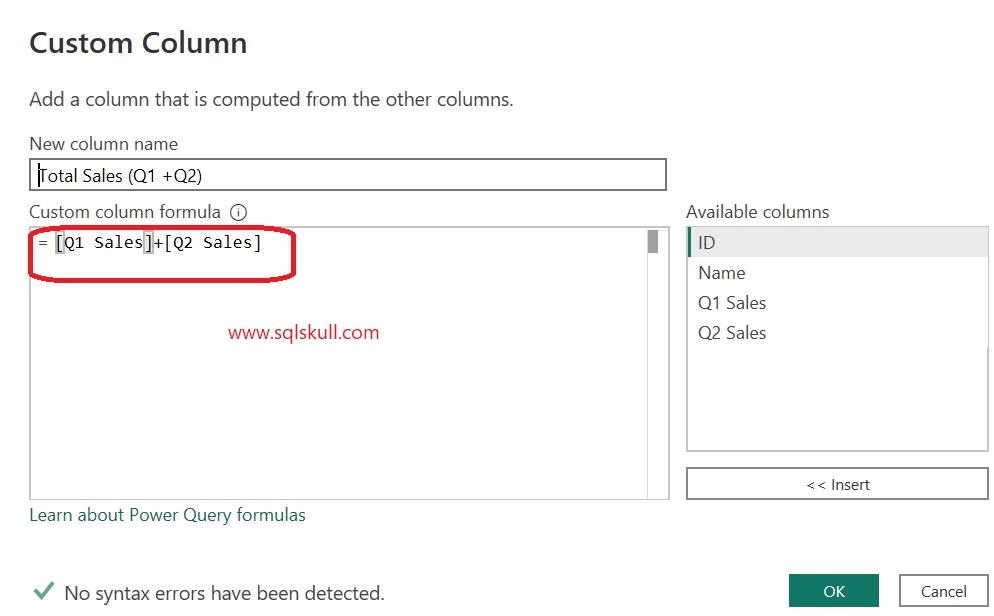But when you look at the result, you can see it returns NULL wherever either of Q1 or Q2 sales have null values.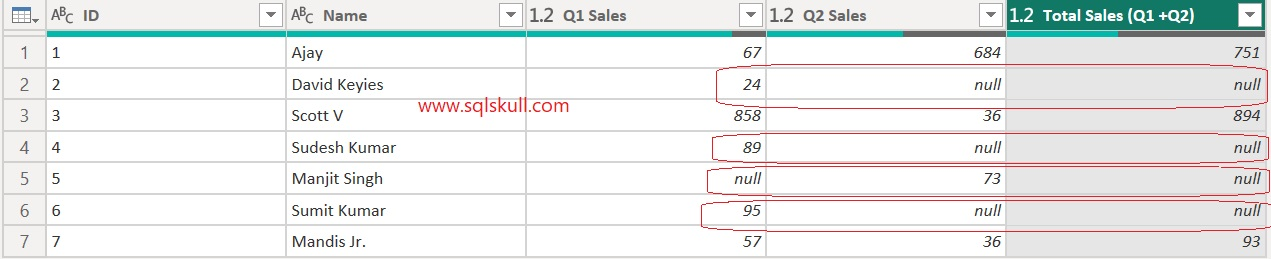It happens because sum operator does not work with NULL values so when you add any value with NULL it returns NULL.

For example, Id =2, the Q1 Sales is 24 while Q2 sales is null. So the Total Sales (Q1 +Q2) will be 24 + NULL that is NULL while the final result should be 24.

Let’s see how to handle NULL values while performing calculation

#### Replace NULL values with zero

You can replace NULL values with Zero, for this go to Power Query Editor then right click on column header (Q1 Sales) after that select Replace Values.. from context menu as shown below.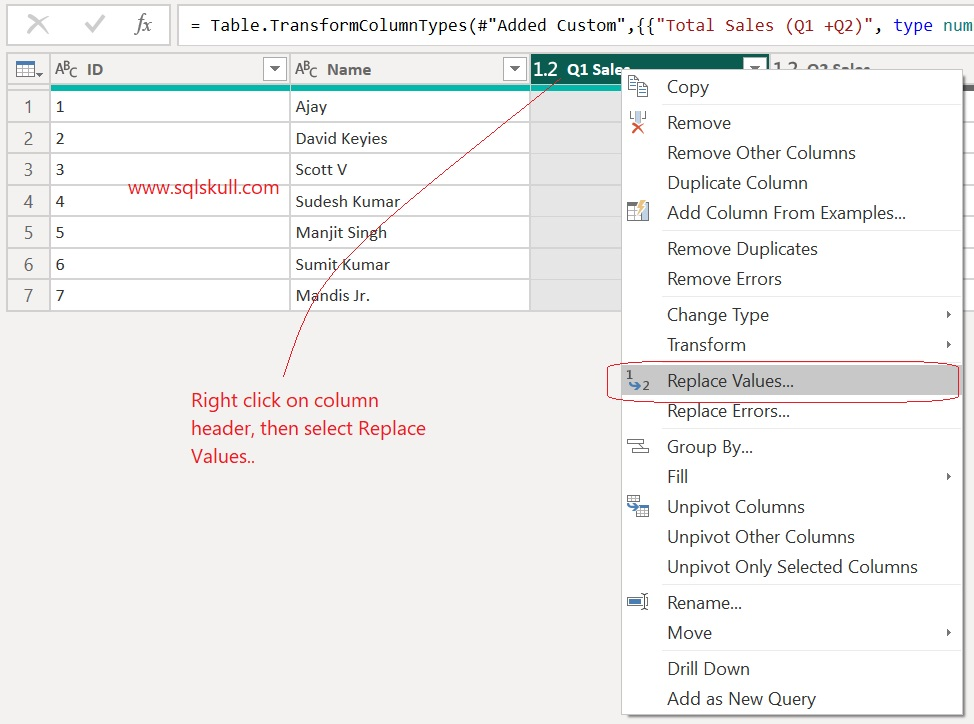Next, a pop window appears here you can replace null values with any value that you want. We replace Null values with zero in our case as shown below.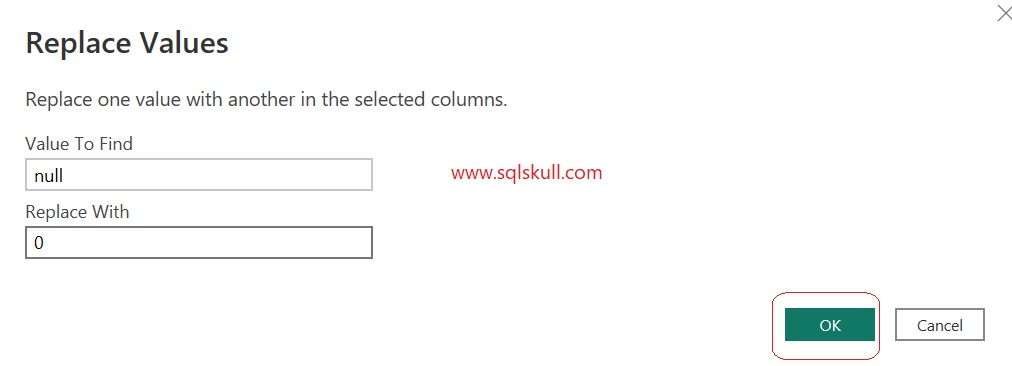Once you done with this, you can see the Null values in Q1 sales are replaced with Zero.Now, repeat the same step for Q2 sales.

Once you replace the NULL values for both Q1 and Q2 sales column.

You can see, now Total sales (Q1+Q2) column returns the correct result.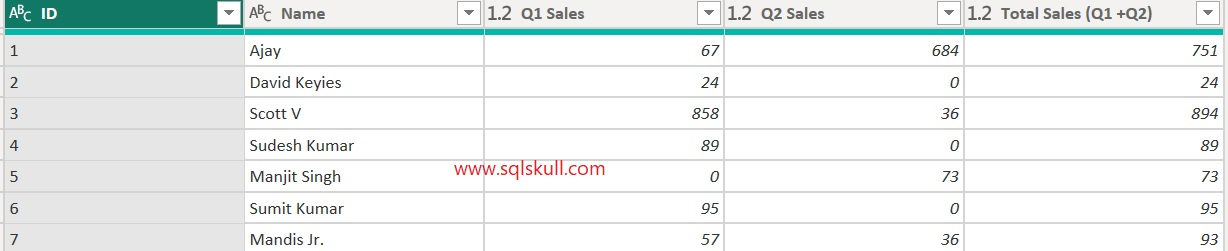#### Replace NULL values using COALESCE operator

You can use COALESCE operator to handle null values, it allows you to return any value that you want if the value in column is NULL. COALESCE operator is represent by double question mark ( ?? ).

Let’s remove all the transformation that you have done so far and get back to base table as shown below.Let’s see, how we can get Total Sales (Q1+Q2) using COALESCE operator.

It handles NULL values by replacing them with zero in real time.

Total Sales (Q1 +Q2)= ( [Q1 Sales] ?? 0) + ([Q2 Sales] ?? 0)You can see, it returns correct result.

Note that, you can see Q1 sales and Q2 Sales column still have NULL values yet it returns correct result because we have used COALESCE operator in our calculation to handle null values.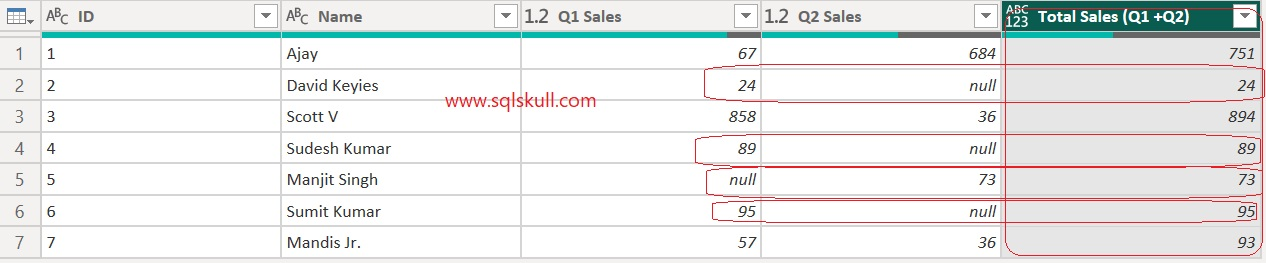#### Replace NULL values using IF statement

You can simply create custom column which uses IF statement to handle NULL values in column. It checks if there is NULL value in column then use 0 otherwise value.

You can write the M code as shown below.

```Total Sales (Q1 +Q2) = (if [Q1 Sales] = null then 0 else [Q1 Sales] ) +
(if [Q2 Sales] = null then 0 else [Q2 Sales])```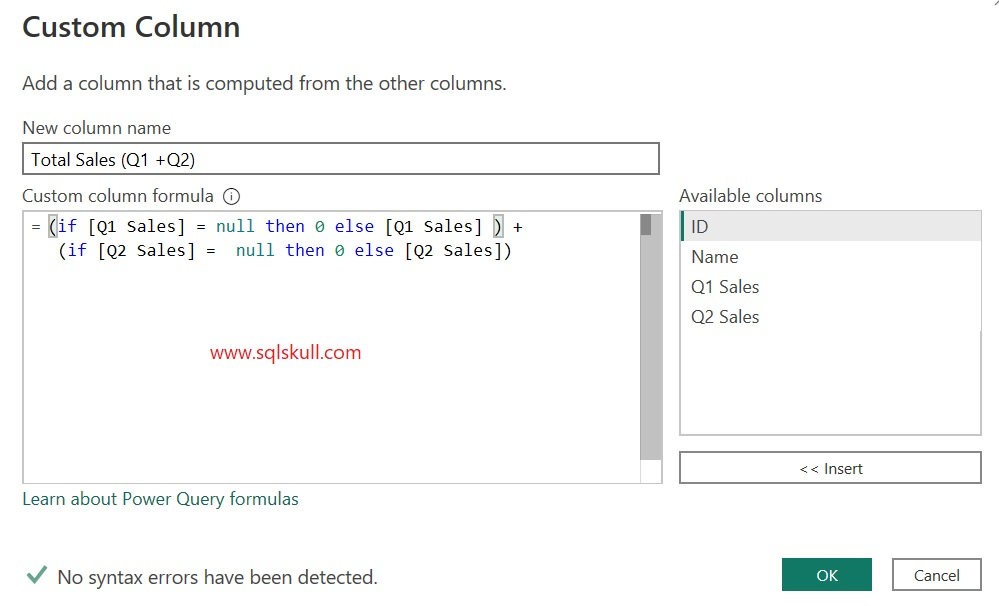It returns the same result.Replace multiple columns values in Power Query

Power Query Tutorial

Power BI DAX Tutorial

Learn SQLThis site uses Akismet to reduce spam. Learn how your comment data is processed.